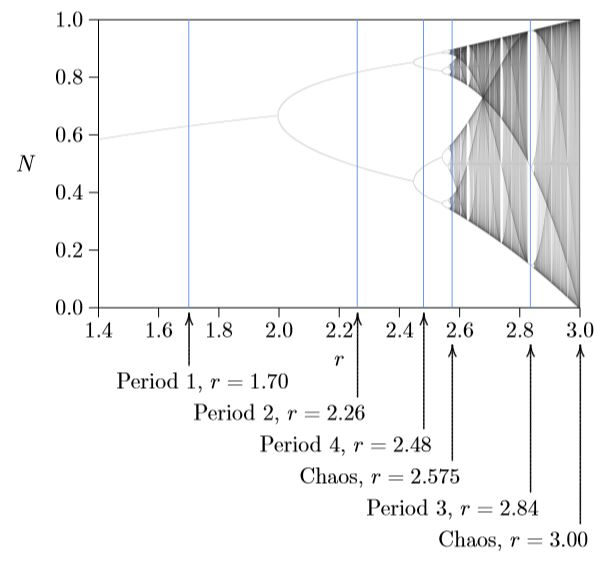$$\newcommand{\vecs}{\overset { \rightharpoonup} {\mathbf{#1}} }$$ $$\newcommand{\vecd}{\overset{-\!-\!\rightharpoonup}{\vphantom{a}\smash {#1}}}$$$$\newcommand{\id}{\mathrm{id}}$$ $$\newcommand{\Span}{\mathrm{span}}$$ $$\newcommand{\kernel}{\mathrm{null}\,}$$ $$\newcommand{\range}{\mathrm{range}\,}$$ $$\newcommand{\RealPart}{\mathrm{Re}}$$ $$\newcommand{\ImaginaryPart}{\mathrm{Im}}$$ $$\newcommand{\Argument}{\mathrm{Arg}}$$ $$\newcommand{\norm}{\| #1 \|}$$ $$\newcommand{\inner}{\langle #1, #2 \rangle}$$ $$\newcommand{\Span}{\mathrm{span}}$$ $$\newcommand{\id}{\mathrm{id}}$$ $$\newcommand{\Span}{\mathrm{span}}$$ $$\newcommand{\kernel}{\mathrm{null}\,}$$ $$\newcommand{\range}{\mathrm{range}\,}$$ $$\newcommand{\RealPart}{\mathrm{Re}}$$ $$\newcommand{\ImaginaryPart}{\mathrm{Im}}$$ $$\newcommand{\Argument}{\mathrm{Arg}}$$ $$\newcommand{\norm}{\| #1 \|}$$ $$\newcommand{\inner}{\langle #1, #2 \rangle}$$ $$\newcommand{\Span}{\mathrm{span}}$$Figure $$\PageIndex{1}$$. Bifurcation diagram summarizing the discrete logistic equation.
The dynamics of all possible values of r can summarized in a “bifurcation diagram” (Figure $$\PageIndex{1}$$). In mathematical terminology, a bifurcation is a place where a tiny change in a parameter causes an extensive and discontinuous change in the behavior of the system. Figure $$\PageIndex{1}$$ shows this by amalgamating the distributions on the right in Figures 7.3.1 through 7.4.2, plus distributions for all other possible values of r. Shading shows where the population spends most of its time. Starting at the right of this figure, fully in the domain of chaos, and moving to the left by reducing r, the behavior moves in and out of chaos-like patterns that never repeat and thus have no period, and also hits stable patterns of every possible period from one up toward infinity.